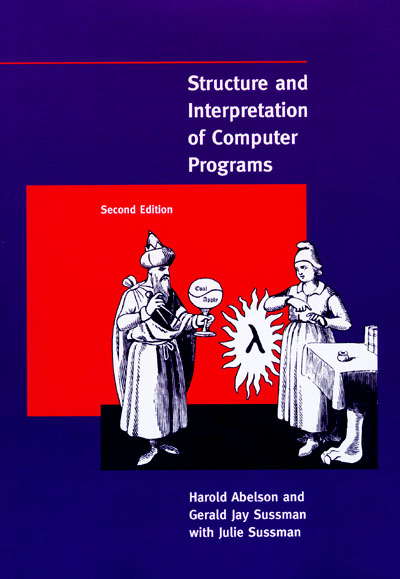# 抽象• 您是否可以把一个多级的系统分类，理解为一颗树，然后用树或图的方式来处理它？
• 如果让您解八皇后问题，或者走一个简单的迷宫，基本上不太会难倒你，但是您可以把自己的思路使用程序表现出来吗？
• 领域驱动设计的一个重要部分，便是将真实世界中的“领域”提炼成模型，再使用计算机语言实现出来。

Thus, when we describe a language, we should pay particular attention to the means that the language provides for combining simple ideas to form more complex ideas. Every powerful language has three mechanisms for accomplishing this:

• primitive expressions, which represent the simplest entities the language is concerned with,
• means of combination, by which compound elements are built from simpler ones, and
• means of abstraction, by which compound elements can be named and manipulated as units.

# 函数式编程

``````static int Sum(int a, int b)
{ int sum = 0; for (int i = a; i <= b; i++) sum += i; return sum;
}``````

``````static int Sum(Func<int, int> f, int a, int b)
{ int sum = 0; for (int i = a; i <= b; i++) sum += f(i); return sum;
}``````

``int i = Sum(x => x * x, 1, 3); // 14 int j = Sum(x => Math.Abs(x), -3, 3); // 12``

``````static Func<int, int, int> SumCurry(Func<int, int> f)
{ return (a, b) => Sum(f, a, b);
}``````

``var sumOfSquare = SumCurry(x => x * x); // int i = sumOfSquare(1, 3); var sumOfCube = SumCurry(x => x * x * x); // int j = sumOfCube(1, 3);``

http://blog.zhaojie.me/2009/07/recommended-reading-2-sicp.html#comment_lkmSMbIA07M00001有兴趣的可以多关注关注这位大牛的博客### loda0128

2017/04/01
0
0

tangchangjie
2013/06/27
0
0

2011/05/01
11K
23

zhangkaixuan
2011/03/19
967
4

2011/03/07
3.6K
25

OSChina 周六乱弹 —— 早上儿子问我他是怎么来的

Osc乱弹歌单（2019）请戳（这里） 【今日歌曲】 @凉小生 ：#今日歌曲推荐# 少点戾气，愿你和这个世界温柔以待。中岛美嘉的单曲《僕が死のうと思ったのは (曾经我也想过一了百了)》 《僕が死の...

1K
13
Excption与Error包结构，OOM 你遇到过哪些情况，SOF 你遇到过哪些情况

Throwable 是 Java 中所有错误与异常的超类，Throwable 包含两个子类，Error 与 Exception 。用于指示发生了异常情况。 Java 抛出的 Throwable 可以分成三种类型。 被检查异常（checked Exc...

Garphy

38
0

FAT_mt

40
0

61
0
tabel 中含有复选框的列 数据理解

1、el-ui中实现某一列为复选框 实现多选非常简单: 手动添加一个el-table-column，设type属性为selction即可； 2、@selection-change事件：选项发生勾选状态变化时触发该事件 <el-table @sel...

everthing

20
0